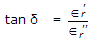# Materials and Components Section 1

26.  The attraction between the nucleus and valence electron of copper atom is
 A. zero B. weak C. strong D. either zero or strong

Explanation:

The valence electron, in copper atom, can be easily detached from nucleus.

27.  If the temperature of an extrinsic semiconductor is increased so that the intrinsic carrier concentration is doubled, then
 A. the majority carrier density is doubled B. the minority carrier density is doubled C. the minority carrier density becomes 4 times the original value D. both the majority and minority carrier densities double

Explanation:

As the intrinsic carrier concentration is doubled, the concentration of both electrons and holes is doubled.

28.  There is no hysteresis phenomenon is any dielectric material.
 A. True B. False

Explanation:

Hysteresis phenomenon exists in dielectic materials.

29.  The units of μ0 and μr are
 A. H/m for both B. H/m for μr and no units for μ0 C. H/m for μ0 and no units for μr D. Wb/m for μ0 and no units for μr

Explanation:

μr is only a numeric.

30.  In metals the valence electron wave functions are strongly perturbed by the presence of neighbouring atoms.
 A. True B. False

Explanation:

In a metal valence electrons are shared by all atoms.

31.  For a paramagnetic material, susceptiblity increases with increasing temperature.
 A. True B. False

Explanation:

Susceptibility ∝.

32.  Hydrogen is used in
 A. large size transformers B. MCB C. large size generators D. circuit breakers

Explanation:

Modem large size generators have hydrogen cooling.

33.  Assertion (A): Ferroelectric materials have spontaneous polarization.

Reason (R): Above curie temperature, ∈ =for ferro-electric materials.

 A. Both A and R are true and R is correct explanation of A B. Both A and R are true but R is not correct explanation of A C. A is true but R is false D. A is false but R is true

Explanation:

Both A and R are correct. Spontaneous polarization vanishes above ferroelectric curie temperature.

34.  If e is the charge of an electron, R is the radius of its orbit and ω is the angular velocity of electron, the magnetic dipole moment μm of the orbit is |μm| = eωR2.
 A. True B. False

Explanation:

1 μm 1 = 0.5 eωr2.

35.  If ∈r and ∈r are the real and imaginary parts of complex dielectric constant ∈r, and δ is the loss angle. Then
 A.B.C. tan δ = ∈‘∈“ D. tan δ = 1 – ∈‘